×
Get Full Access to Penn State - PHYS 211 - Study Guide - Midterm
Get Full Access to Penn State - PHYS 211 - Study Guide - Midterm

×

PENN STATE / Physics / PHYS 211 / What is contact force?

# What is contact force? Description

##### Description: This study guide covers all of Exam 2 material and goes over a lot of practice problems that will be extremely helpful for the exam.
11 Pages 21 Views 20 Unlocks
Reviews

PHYS 211 Midterm Study Guide

## What is contact force?Study guide is most useful with my notes that give highly important diagrams I will also skip useless and unimportant subchapters so you save a lot of time studying from my study guide. This study guide highlights only the important parts of my notes, so you save time​. ____________________________________________________________________________

Equations to know how to use​ (a lot, but most are similar and easy to understand): 1. Fnet = ma

2. Fg = mg

3. Fg = (GmM)/r2

4. Fs = usn

## What is a long-range force?5. Fk = ukn

6. Fr = urn

7. Fd = -½CpAv2

8. ac = v2/r = w2r

9. T = 2pi/w = 2pi*r/v = 1/f We also discuss several other topics like kensha clark

10. Fs = Static Friction

11. Fk = Kinetic Friction

12. Fr = Rolling Friction

13. Fg = Force of Gravity If you want to learn more check out pols2401

G = 6.67x10-11 Nm2/kg2

## What is a mass?Fd = Drag Force

T = Tension Force

Ft = Thrust Force

ac = Centripetal acceleration (acceleration towards the center)

T = Period

w = Angular velocity

v = Linear Velocity

f = Frequency

Know unit circle (easy points on easy questions)

____________________________________________________________________________

Chapter 5 – Force and Motion ​(mostly definitions and explanation, not important chapter) 5.1-Force:

Force- Acts on an object

Agent- Something that exerts a force

Contact Force- Two objects touching

Long Range Force- Forces acting on each other without physical contact Force Examples: Don't forget about the age old question of example of cultural particulars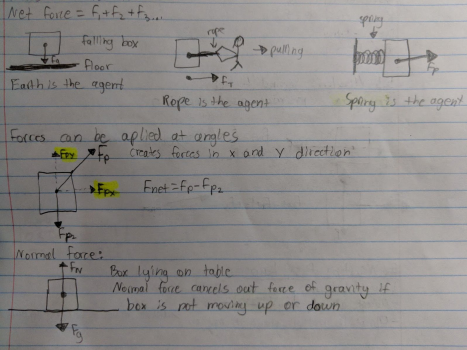Friction:

1. Force parallel to surface

2. If it keeps object stationary = static friction (Fs)

3. If it opposes motion = kinetic friction (Fk)

5.5-Newton’s Second Law:

Fnet = ma We also discuss several other topics like materiality constraint

m = mass

a = acceleration

5.6-Newton’s First Law:

Law of inertia:

1. Object at rest, stays at rest unless acted upon by an outside Force

2. Object in motion, stays in motion unless acted upon by an outside Force

*Objects accelerations do not change unless a new force is added

If Fnet = 0 that object has:

● Constant velocity

○ That object’s velocity could be +, -, or 0

● Acceleration = 0

Inertial Reference Frame- When Fnet = 0 an object is an inertial reference frame, because it is not accelerating

____________________________________________________________________________ Chapter 6 – Motion Along a Line Don't forget about the age old question of emily indriolo

6.1-Equilibrium Model:

Objects with a net force of 0 (Fnet = 0) have NO acceleration

Objects in Equilibrium Examples: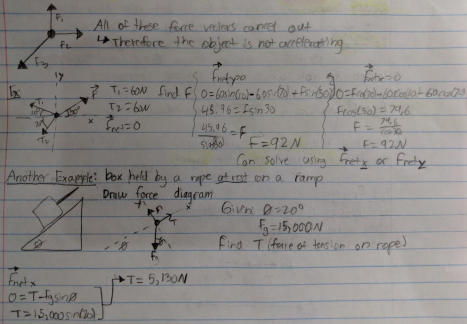6.2-Using Newton’s Second Law: We also discuss several other topics like imotu

The forces acting on an object determine its acceleration

Fnet = ma

We can use this and our past knowledge from other chapters to solve for Vffrom Vi and Fnet Examples ​(next page):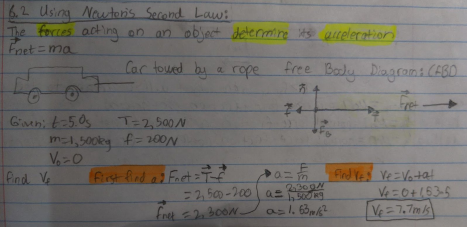6.3- Mass, Weight, and Gravity:

Mass is unchanging (mass = how much “stuff is in an object”)

An object’s weight depends upon the gravity of where it is (less on the moon than on Earth) Gravity- Long range attractive force between two objects (Fg = (GmM)/r2) m = mass of smaller object

M = mass of larger object

*Gravity gets weaker as distance increases

Gravity on an object on Earth = FEarth = mg (straight down)

*Something will weigh more while accelerating up (in an elevator), but mass will stay the same

6.4-Friction:

Static Friction:

1. Force on an object that keeps it from moving

2. Matches a force trying to move an object, until it is overcome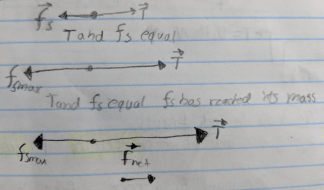6.5 Drag ​(not important subchapter, but just know what the formula means): Drag Coefficient- Tells how strong of a force is going against motion

Fd = -½CpAv2in the direction opposite of motion

A = Area of object

p = density of air (1.3 kg/m3 at 0oC)

C = Drag coefficient (smaller for more aerodynamic objects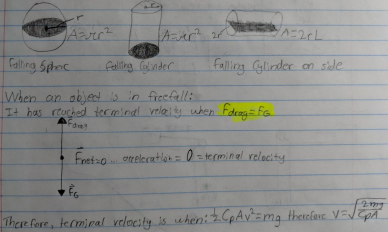____________________________________________________________________________ Chapter 7 – Newton’s Third Law

7.1-Interacting Objects:

Any time an object, A, pushed or pulls on another object, B, then that object, B, pushes or pulls back on A

Every force is part of an action reaction pair:

-Earth pulls down on you

-You pull up on the Earth

Example​ (next page):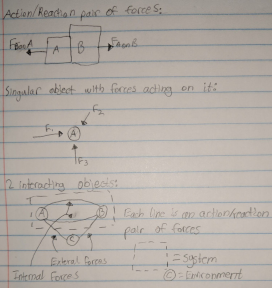*When walking, what force makes you move forward?

Ans:​ The Earth pushing forward against you (because you push back on it) 7.3-Newton’s Third Law:

As stated before objects A and B exert a force on each other

-The Force of A on B is equal to Force B on A

-These two Forces are in opposite directions

Ex​ (stated before):

The Earth pulls down on you, and you pull up on the Earth with equal Force Thought Question:

A car is pushing a truck. The mass of the truck is much larger than the mass of the car. What can be said about the forces between the two?

Ans:​ The car exerts the same amount of force on the truck as the truck exerts on the truck - Equal Force and opposite direction

7.4-Ropes and Pulleys​ (many practice problems):

Tension Forces- in ropes is causing by the stretching of their “spring-like molecular bonds” -Don’t remember that...it’s useless

-Just know Tension is a force applied by a rope or pulley!!!

Practice Problems​ (next page and answers follow picture or are in picture):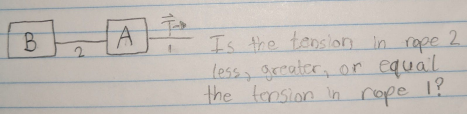Ans:​ Less than (rope 1 has to pull the weight of A and B, whereas 2 only pulls the weight of B)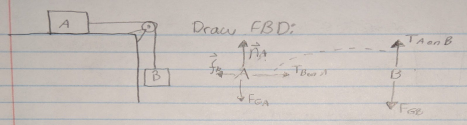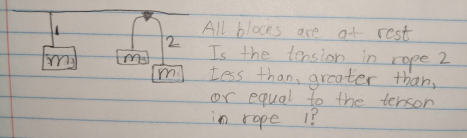Ans:​ Equal to (both ropes experience a force of tension equal to mg)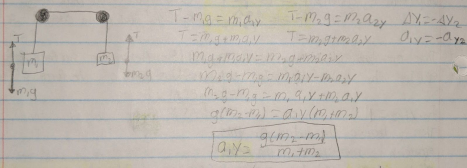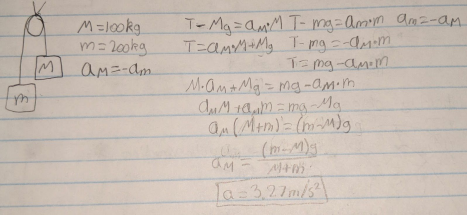____________________________________________________________________________ Chapter 8 – Dynamic II: Motion in a Plane:

8.1-Dynamics in Two Dimensions:

Linear motion and Two-Dimensional motion follow the same rules of Fnet = ma Example of Two Dimensions:

A Rocket Launching upwards with wind pushing it. How far does the wind push it? Rocket Mass = 30 kg

Thrust Force = 1500 N

Wind Force = 20 N

Distance up = 1,000 m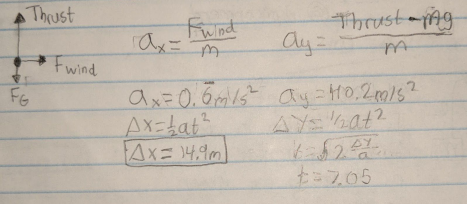How will this Force affect the object’s momentum: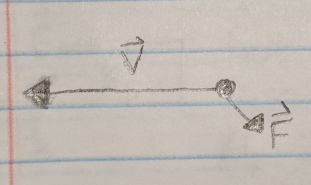Ans:​ It slows down, and curves downward

8.2-Uniform Circular Motion:

ac = v2/r = w2r

That is an old equation, but now we have to know how forces cause this acceleration Therefore we can say:

Fnet = mac = mv2/r = = mw2r

Example of Formula Use​ (picture on next page):

Question: A father puts his 20 kg child in a 5 kg cart, and swings him with a 2 meter rope creating a tension of 100 N. What is the RPM of the cart?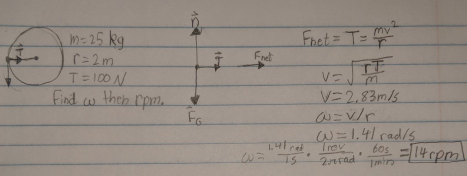What is the maximum speed a 1,500 kg car can make a left turn around a curve of 50 m without sliding? (Static friction coefficient is 1)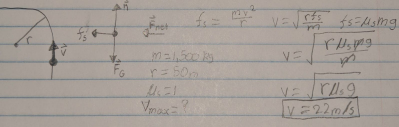A highway curve of radius 70 m is banked at an angle of 15 degrees. At what speed can a car take this bank without assistance from friction?

A Stone Age hunter swings a 1 kg rock around his head with a 1 m rope. If the rope breaks at a Tension of 200 N, what is the max angular speed, in rpm, he can swing the rock?

8.5-Nonuniform Circular Motion:

Objects are in Nonuniform Circular Motion when they are following a circular path, and its speed is changing

- Along with centripetal acceleration, there is tangential acceleration

-Remember v is positive when counterclockwise and negative when clockwise -Force follows the same rules

A 1,500 kg car is going around a 25 m radius track, starting from rest. The wheels supply a constant 525 N Force in the forward direction. The coefficient of static friction between the tires and road is 0.9. How many revolutions have been made when the car slides off the track?

Page ExpiredIt looks like your free minutes have expired! Lucky for you we have all the content you need, just sign up here
References: